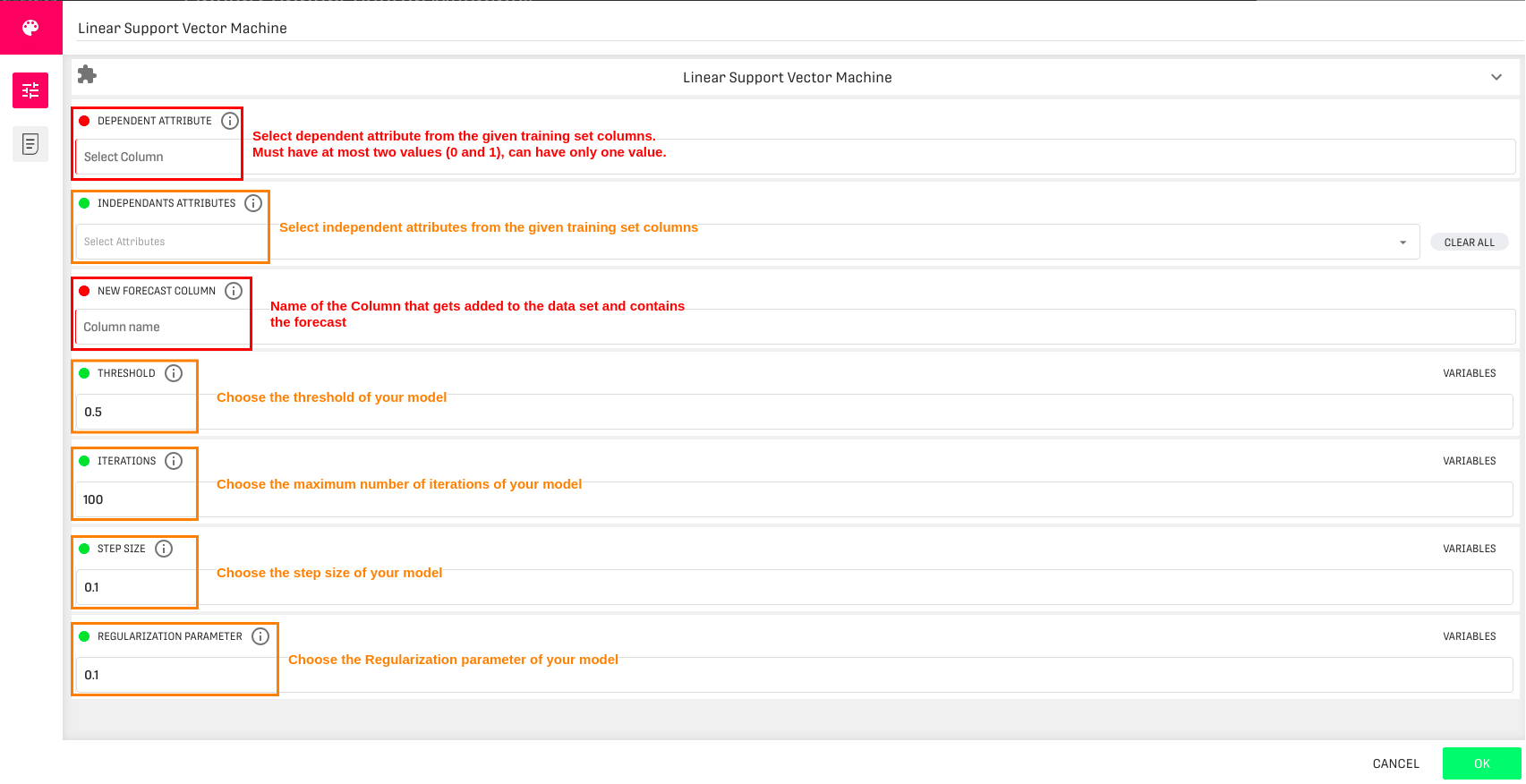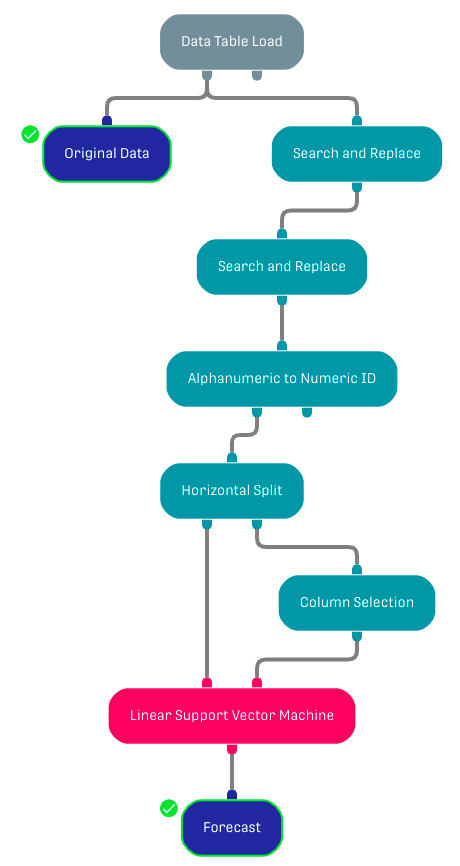# Overview

The Linear Support Vector Machine Processor is used for binary classification. Linear SVM is a standard method for large-scale classification tasks.

# Input

The processor requires two input datasets. The left input port corresponds to the training dataset (this data should be already labeled). The right input port corresponds to the test dataset (target columns should be of numeric type, target classes should have values 0 and 1).

The training and the test datasets must have the same schema.

# ConfigurationNote that dependent and independent variables need to be of numeric type.

# Output

The processor returns the input test dataset with an additional column containing the forecasted values.

# Example

## WorkflowThe columns with binary values in the original dataset have 'Male' or 'Female' string values. Two Search and Replace processors are used to replace 'Male' with '1' and 'Female' with '0'. Then the Alphanumeric to Numeric ID processor is used to transform these string numbers to integers. The dataset is split into a training and test dataset and inserted in the target processor by a Horizontal Split processor (only the dependent and independent columns are selected in the test dataset using the Column Selection processor).

# Further Information

To check the outcome of your model you can create a forecast metric using a Query Processor. The SQL command is as follows:

```SELECT count(*) correct, 0 incorrect FROM inputTable
WHERE [dependent variable] = [new forecast column] UNION (
SELECT 0 correct, count(*) incorrect FROM inputTable
WHERE [dependent variable] <> [new forecast column]
)```

The result will be presented in a Result Table Processor.

The Multiclass Linear Support Vector Machine Processor works similarly and is not restricted to binary classification.

# Related Articles

Decision Tree Regression Forecast

Decision Tree Classification Forecast

Random Forest Classification Forecast Processor

Random Forest Regression Forecast Processor